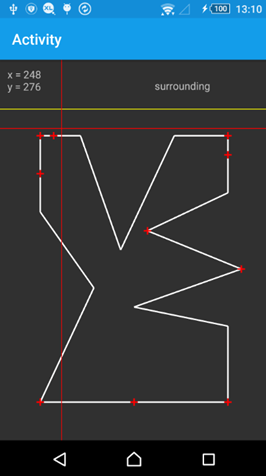# Android QuestionPolygon calculation

#### Duncan williamson

##### Member
Longtime User
Hi, im using googlemapextras and have a series of polygons which define property boundaries.
I need to be able to calculate if a given lat/lng is within a specified distance of the edge of any part of the polygon..
I have seen code posted which shows if a given lat/lng is within a polygon or outside but cannot see how to modify it to check distance... wondering if an expert can point me in the right direction

regards
Duncan

#### klaus

##### Expert
Longtime User
Attached you find a small project which returns if a point is inside the polygon, in the surrounding with a given distance or outsides the surrounding.
It is an evolution of this project.
The routine checks first if the point is in the polygon and then check if the distance of the point to the each polygon line is smaller than the given distance.
The equations are general graphic equations not exactly for maps.
But as Erel alrady pointed out: If the polygon is not too large then you can ignore the fact that the coordinates system is not Euclidean

The red lines show the cursor.
The yellow line shows the surrounding distance used (shown only on one side).The coordinates of the polygon vertexes are saved in two arrays of Doubles.
For lat/long coordinates you could calculate the distance like this.
DistanceDegrees = DistanceKiloMeters / 6371 * 180 / cPi
DistanceDegrees = DistanceMiles / 3959 * 180 / cPi

#### Attachments

• PointInPolygonSurrounding.zip
9.2 KB · Views: 262

#### Duncan H Williamson

##### Member
Longtime User
Attached you find a small project which returns if a point is inside the polygon, in the surrounding with a given distance or outsides the surrounding.
It is an evolution of this project.
The routine checks first if the point is in the polygon and then check if the distance of the point to the each polygon line is smaller than the given distance.
The equations are general graphic equations not exactly for maps.
But as Erel alrady pointed out: If the polygon is not too large then you can ignore the fact that the coordinates system is not Euclidean

The red lines show the cursor.
The yellow line shows the surrounding distance used (shown only on one side).

View attachment 42597

The coordinates of the polygon vertexes are saved in two arrays of Doubles.
For lat/long coordinates you could calculate the distance like this.
DistanceDegrees = DistanceKiloMeters / 6371 * 180 / cPi
DistanceDegrees = DistanceMiles / 3959 * 180 / cPi

Thank you Klaus
I really appreciate all the expertise and help forwarded by everyone on this forum

Regards

Duncan

#### wonder

##### Expert
Longtime User
Attached you find a small project which returns if a point is inside the polygon, in the surrounding with a given distance or outsides the surrounding.
It is an evolution of this project.
The routine checks first if the point is in the polygon and then check if the distance of the point to the each polygon line is smaller than the given distance.
The equations are general graphic equations not exactly for maps.
But as Erel alrady pointed out: If the polygon is not too large then you can ignore the fact that the coordinates system is not Euclidean

The red lines show the cursor.
The yellow line shows the surrounding distance used (shown only on one side).

View attachment 42597

The coordinates of the polygon vertexes are saved in two arrays of Doubles.
For lat/long coordinates you could calculate the distance like this.
DistanceDegrees = DistanceKiloMeters / 6371 * 180 / cPi
DistanceDegrees = DistanceMiles / 3959 * 180 / cPi
This is AWESOME for game development!!! Thank you so much!!!Replies
2
Views
411
Replies
6
Views
316
Android Question Draw a Polygon on a Map
Replies
4
Views
911
Replies
25
Views
9K
Replies
13
Views
2K